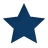# Dynamic Geometry & Circle TheoremsThese pages have a page with a dynamic geometry window for each of the eight circle theorems. You can use them to work out what the theorems are. I've also done proofs for five of the theorems, and activities (which involve paper, pencil &/or scissors) for two of them. The Activities: First circle theorem - angles at the centre and at the circumference. Third circle theorem - angles in the same segment. The dynamic geometry pages: First circle theorem - angles at the centre and at the circumference. Second circle theorem - angle in a semicircle. Third circle theorem - angles in the same segment. Fourth circle theorem - angles in a cyclic quadlateral. Fifth circle theorem - length of tangents. Sixth circle theorem - angle between circle tangent and radius. Seventh circle theorem - alternate segment theorem. Eighth circle theorem - perpendicular from the centre bisects the chord The proofs: First circle theorem - angles at the centre and at the circumference. Second circle theorem - angle in a semicircle. Third circle theorem - angles in the same segment. Fourth circle theorem - angles in a cyclic quadlateral. Seventh circle theorem - alternate segment theorem. (On each proof page there is a link to a downloadable pdf version)

Resource Type
Activity
Tags
activities  circle  discover  proofs  theorem  theorems
Target Group (Age)
14 – 18
Language
English (United Kingdom)

• GeoGebra
• Help
• Partners